# DC Pandey Solutions: Modern Physics I - 1 Notes | Study DC Pandey Solutions for JEE Physics - JEE

## JEE: DC Pandey Solutions: Modern Physics I - 1 Notes | Study DC Pandey Solutions for JEE Physics - JEE

The document DC Pandey Solutions: Modern Physics I - 1 Notes | Study DC Pandey Solutions for JEE Physics - JEE is a part of the JEE Course DC Pandey Solutions for JEE Physics.
All you need of JEE at this link: JEE

Introductory Exercise 30.1

Ques 1: The wavelength for n = 3 to n = 2 transition of the hydrogen atom is 656.3 nm. What are the wavelengths for this same transition in
(a) positronium, which consists of an electron and a positron
(b) singly ionized helium (Note: A positron is a positively charged electron).
Sol:
(a) Reduced mass of positronium and electron is m/2 where m = mass of electron)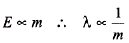m has become half, so λ will become two times or 1312 nm or 1.31 nm.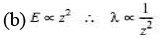For singly ionized helium atom z = 2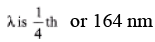Ques 2: Find the longest wavelength present in the Balmer series of hydrogen.
Sol:
Longest wavelength, means minimum energy.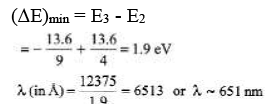Ques 3: (a) Find the frequencies of revolution of electrons in n = 1 and n = 2 Bohr orbits. (b) What is the frequency of the photon emitted when an electron in an n = 2 orbit drops to an n = 1 hydrogen orbit?
(c) An electron typically spends about 10-8s in an excited state before it drops to a lower state by emitting a photon. How many revolutions does an electron in an n = 2 Bohr hydrogen orbit make in 1.00 × 10-8 s?
Sol: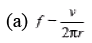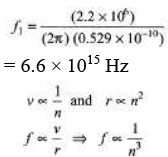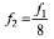(b) ΔE =E2 - E1 = 10.2 eV = hf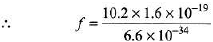= 6.47 × 1015Hz
(c) In option (a), we have found that,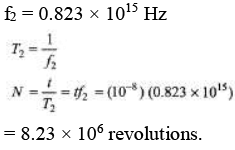Ques 4: A muon is an unstable elementary particle whose mass is 207 me and whose charge is either +e or-e. A negative muon (μ-) can be captured by a nucleus to form a muonic atom.
(a) A proton captures a μ-. Find the radius of the first Bohr orbit of this atom.
(b) Find the ionization energy of the atom.
Note Attempt this question after reading the whole chapter.
Sol: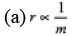∴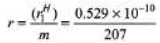= 2.55 × 10-13 m
(b) E ∝ m
∴ Ionization energy of given atom =
(m) (ionization energy of hydrogen atom)
= (207) (13.6 eV) = 2815.2 eV= 2.81 keV

Ques 5: Find the de-Broglie wavelengths of
(a) a 46 g golf ball with a velocity of 30 m/s,
(b) an electron with a velocity of 107 m/s.
Sol: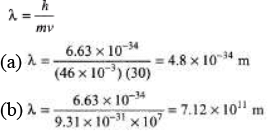Ques 6: (a) A gas of hydrogen atoms in their ground state is bombarded by electrons with kinetic energy 12.5 eV. What emitted wavelengths would you expect to see?
(b) What if the electrons were replaced by photons of same energy?
Sol:
(a) En -E1 = 12.5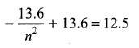Solving we get, n = 3.51
Hence electron jumps to n = 3.
So possible lines are between n = 3 to n = 2, n = 3 to n = 1 and between n = 2 to n = 1.
For n = 3 to n = 2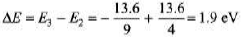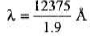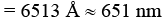Similarly other wavelengths can also be obtained.
(b) n = 3.51 (in option-a)
A photon always transfers its energy completely.
So it cannot excite the ground state electrons to n = 3 (like and electrons excited it in part-a).

Ques 7: For a given element the wavelength of the Kα line is 0.71 nm and of the Kβ line it is 0.63 nm. Use this information to find wavelength of the Lα line.
Sol: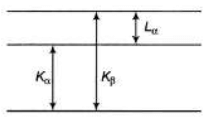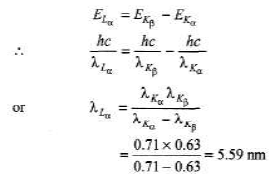Ques 8: The energy of the n = 2 state in a given element is E2 = - 2870 eV. Given that the wavelengths of the Kα and Kβ lines are 0.71 nm and 0.63 nm, respectively determine the energies E1 and E3.
Sol: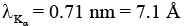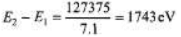E1 =E2 - 1743 = -2870 - 1743 = 4613 eV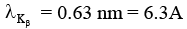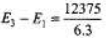= 1964 eV
∴ E3 = E1 + 1964
= -4613+1964 = -2649 eV

Ques 9: The energy levels of a certain atom are shown in figure. If a photon of frequency f is emitted when there is an electron transition from 5E to E, what frequencies of photons could be produced by other energy level transitions?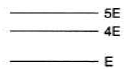Sol:
5E - E = hf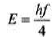...(i)
Between 5E and 4E
5E - 4E = hf1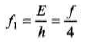(from eq. (i))
Between 4E and E
4E - E = hf2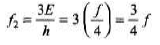The document DC Pandey Solutions: Modern Physics I - 1 Notes | Study DC Pandey Solutions for JEE Physics - JEE is a part of the JEE Course DC Pandey Solutions for JEE Physics.
All you need of JEE at this link: JEEUse Code STAYHOME200 and get INR 200 additional OFF

## DC Pandey Solutions for JEE Physics

210 docs

Track your progress, build streaks, highlight & save important lessons and more!

,

,

,

,

,

,

,

,

,

,

,

,

,

,

,

,

,

,

,

,

,

;